DDG（离散微分几何）：对外微积分快速粗暴的一个介绍 | HyperPlane

• 线性代数：小箭头 如果你曾经学过线性代数，你可能记得有些东西必须使用“小箭头”——也被称为向量。实际上，如果这是你能记起来关于线性代数的所有内容，那么现在是一个绝佳的好时机回去重新学一遍！我们这里将不会复习。
• 向量微积分：小箭头是怎么变化的？ 同样的，如果你学过向量微积分，那么你记得向量微积分必须随着“小箭头”如何在空间和时间上变化操作（例如，风向变化有多快）。换句话说，向量微积分告诉我们如何微分向量。我们也同样不会复习这个话题！
• 外代数：小体积 线性代数探索了很多你可以用向量操作的事情：你可以加它们，你可以数乘它们，你可以进行内积，可以外积，还有其它的操作。外代数仅仅增加了几个操作到这个列表里面，这让讨论像面积和体积这样的东西更简单。特别的，这些操作让我们构造出被称为k-向量（k-vectors）的东西，它可以被当作“小k-维体积（little k-dimensional volumes）”。
• 外微积分：小体积怎么变化？ 最后，如果向量微积分是研究“小箭头”如何在空间和时间上变化的课题，那么外微积分是研究小体积如何在空间和时间上变化的课题。换句话说，外积分告诉我们如何微分k-向量。

## 外代数

（注意：k-向量和k维向量在这里面表示的意义是不一样的）

### 热身：1-向量和2-向量

1. 它们有“有限的大小”，即它们有一个大小
2. 它们有朝向（orientation）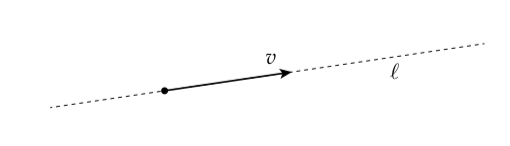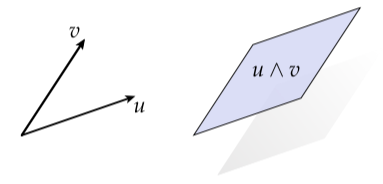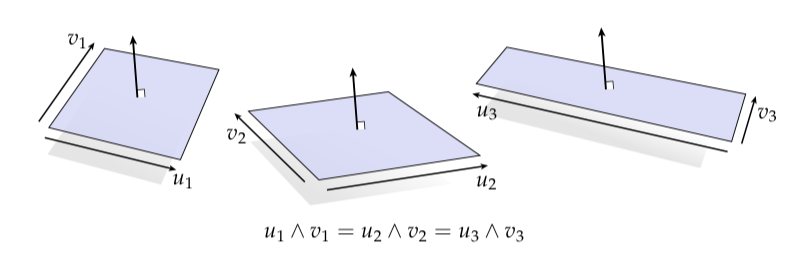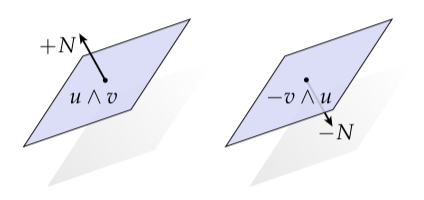$u \wedge u = 0$$(au) \wedge v = a(u \wedge v)$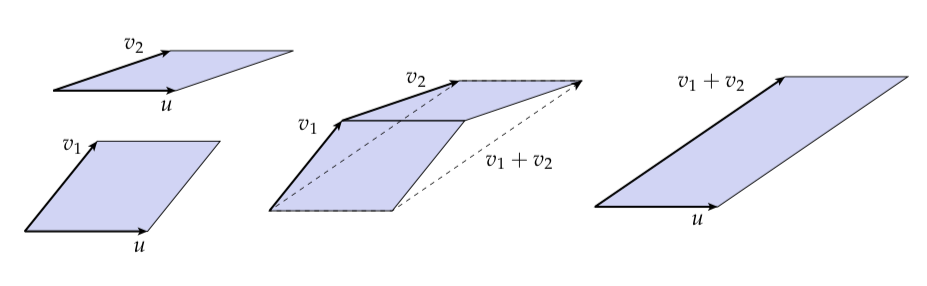$u \wedge v_1 + u \wedge v_2 = u \wedge (v_1 + v_2)$

（你能在3D中构造出类似的图吗？）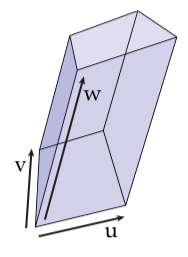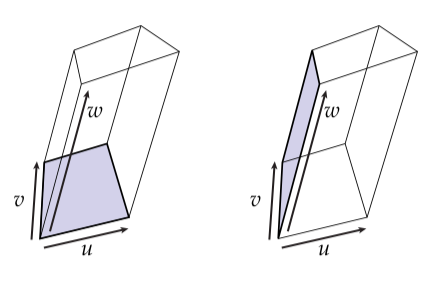$(u \wedge v) \wedge w = u \wedge (v \wedge w)$

$u \wedge v \wedge w = - v \wedge u \wedge w$

$u \wedge v \wedge w = u \wedge w \wedge v = w \wedge u \wedge v$

$w \wedge v \wedge u = v \wedge u \wedge w = u \wedge w \wedge v$

### 楔形积

• 反对称性（Antisymmetry） $u \wedge v = -v \wedge u$
• 结合律（Associativity） $(u \wedge v) \wedge w = u \wedge (v \wedge w)$
• 加法分配律（Distributivity over addition） $u \wedge (v + w) = u \wedge v + u \wedge w$
• 标量乘法分配律（Distributivity of scalar multiplication） $(au) \wedge (bv) = ab(u \wedge v)$

### Hodge星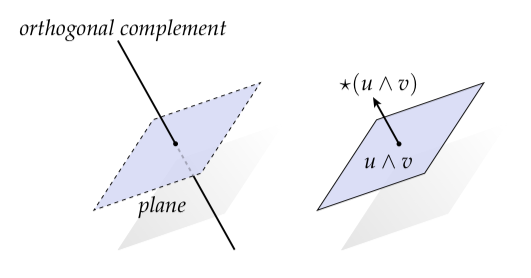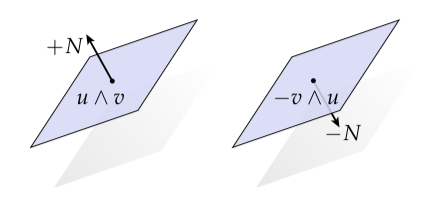$\det (u, v, \star (u \wedge v)) > 0$

$\det (u_1, u_2, \star(u_1 \wedge u_2)) = 1$

$(u_1 \wedge \dots \wedge u_k) \wedge \star (u_1 \wedge \dots u_k) = e_1 \wedge \dots \wedge e_n$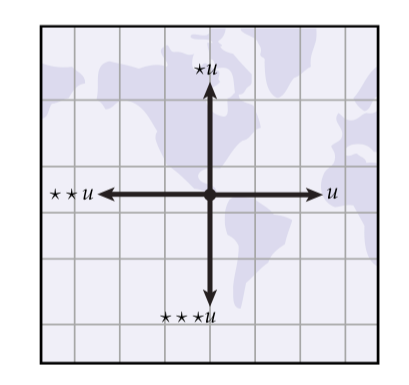$\star (u + v) = \star u + \star v$

## $\mathbb{R}^n$中楔形积和星的例子

\begin{aligned} u \wedge v &= (e_1 + 2e_2) \wedge (e_1 + e_2 - e_3) \\ &= e_1 \wedge(e_1 + e_2 - e_3) + 2e_2(e_1 + e_2 - e_3) \\ &= \cancel{e_1 \wedge e_1}^0 + e_1 \wedge e_2 - e_1 \wedge e_3 + 2e_2 \wedge e_1 + \cancel{2e_2 \wedge e_2}^0 - 2e_2 \wedge e_3 \\ &= e_1 \wedge e_2 - 2e_1 \wedge e_2 - e_1 \wedge e_3 - 2e_2 \wedge e_3 \\ &= -e_1 \wedge e_2 - e_1 \wedge e_3 - 2e_2 \wedge e_3 \end{aligned}

\begin{aligned} \star w &= \star(-e_1 \wedge e_2 - e_1 \wedge e_3 - 2e_2 \wedge e_3) \\ &= -\star(e_1 \wedge e_2) - \star(e_1 \wedge e_3) - 2\star(e_2 \wedge e_3) \\ &= - e_3 -(-e_2) - 2(e_1) \\ &= -2 e_1 + e_2 - e_3 \end{aligned}

\begin{aligned} v \wedge w &= (e_1 + 2e_2 + 3e_3) \wedge (e_1 - e_3) \\ &= \cancel{e_1 \wedge e_1}^0 - e_1 \wedge e_3 + 2e_2 \wedge e_1 - 2e_2 \wedge e_3 + 3e_3 \wedge e_1 - 3\cancel{e_3 \wedge e_3}^0 \\ &= -2 e_1 \wedge e_2 - 4e_1 \wedge e_3 - 2e_2 \wedge e_3 \end{aligned}

$u$进行楔形积然后得到

\begin{aligned} u \wedge (v \wedge w) &= (e_1 + e_2 + e_3) \wedge (-2 e_1 \wedge e_2 - 4 e_1 \wedge e_3 - 2 e_2 \wedge e_3) \\ &= -2 e_1 \wedge e_2 \wedge e_3 - 4 e_2 \wedge e_1 \wedge e_3 - 2 e_3 \wedge e_1 \wedge e_2 \\ &= -2 e_1 \wedge e_2 \wedge e_3 + 4 e_1 \wedge e_2 \wedge e_3 - 2 e_1 \wedge e_2 \wedge e_3 \\ &= 0 \end{aligned}

## 向量和1-型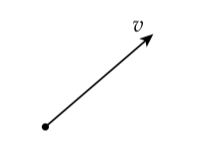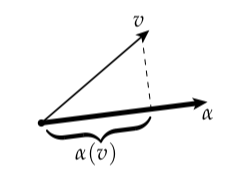$\begin{bmatrix} \alpha_1 & \cdots & \alpha_n \end{bmatrix} \begin{bmatrix} v^1 \\ \vdots \\ v^n \end{bmatrix}$

$v^Tv = \begin{bmatrix} v^1 & \cdots & v^n \end{bmatrix} \begin{bmatrix} v^1 \\ \vdots \\ v^n \end{bmatrix}$

$u^{\flat}(v) = \mathsf{g}(u,v)$

### 坐标系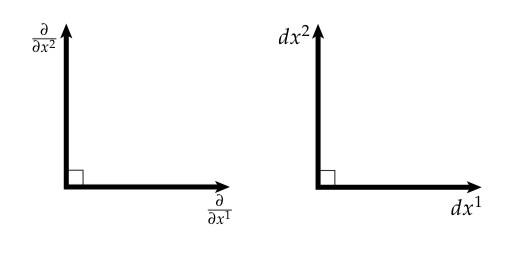$v = v^1 \frac{\partial}{\partial x^1} + \cdots + v^n \frac{\partial}{\partial x^n}$

$\alpha = \alpha_1 dx^1 + \dots + \alpha_n dx^n$

$dx^i \left( \frac{\partial}{\partial x^i} \right) = \delta^i_j = \begin{cases} 1,& i=j \\ 0,& \text{otherwise} \end{cases}$

$\alpha(v) = \sum_i \alpha_i dx^i \left(\sum_j v^j \frac{\partial}{\partial x^j}\right) = \sum_i \alpha_i v^i$

### 符号

$\alpha_i v^i = \sum_i \alpha_i v^i$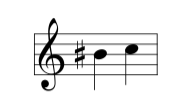$\alpha = \alpha_1 dx^1 + \cdots + \alpha_n dx^n$

$\alpha ^{\sharp} = \alpha^1 \frac{\partial}{\partial x^1} + \cdots + \alpha^n \frac{\partial}{\partial x^n}$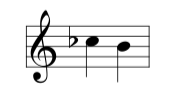$v = v^1 \frac{\partial}{\partial x^1} + \cdots + v^n \frac{\partial}{\partial x^n}$

$v^{\flat} = v_1dx^1 + \cdots + v_ndx^n$

## 微分形式和楔形积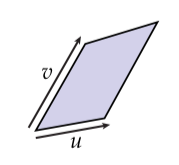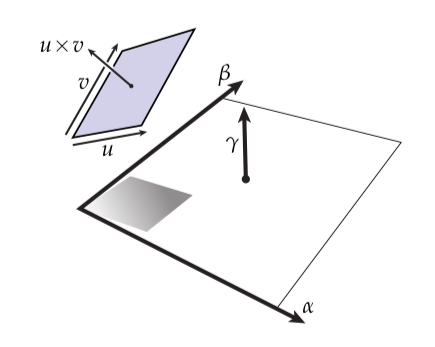\begin{aligned} u' &= (\alpha(u), \beta(u))^T \\ v' &= (\alpha(v), \beta(v))^T \end{aligned}

$u' \times v' = \alpha(u)\beta(v) - \alpha(v)\beta(u)$

$\alpha \wedge \beta (u,v) = \alpha(u)\beta(v) - \alpha(v)\beta(u)$

$\alpha \wedge \beta = - \beta \wedge \alpha$

\begin{aligned} \alpha \wedge \alpha &= - \alpha \wedge \alpha \\ \Rightarrow \alpha \wedge \alpha &= 0 \end{aligned}

\begin{aligned} \alpha \wedge \beta (u,v) + \alpha \wedge \gamma (u,v) &= \alpha(u)\beta(v) - \alpha(v)\beta(u) + \alpha(u)\gamma(v) - \alpha(v)\beta(u) \\ &= \alpha(u)(\beta(v) + \gamma(v)) - \alpha(v)(\beta(u) + \gamma(u)) \\ &= (\alpha \wedge (\beta + \gamma))(u,v) \end{aligned}

$\alpha \wedge (\beta + \gamma) = \alpha \wedge \beta + \alpha \wedge \gamma$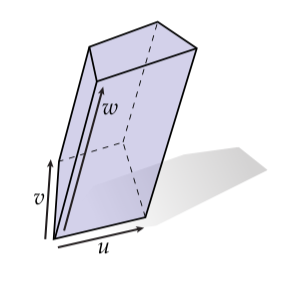$\alpha \wedge \beta \wedge \gamma (u,v,w) \coloneqq \det(\begin{bmatrix} u' & v' & w' \end{bmatrix}) = \det \left( \begin{bmatrix} \alpha(u) & \alpha(v) & \alpha(w) \\ \beta(u) & \beta(v) & \beta(w) \\ \gamma(u) & \gamma(v) & \gamma(w) \end{bmatrix} \right)$

（你注意到了左上角$2 \times 2$子矩阵的行列式也给了我们两个1-型的楔形积吗？）或者说，我们可以将体积解释成一个面的面积乘上剩下边的长度：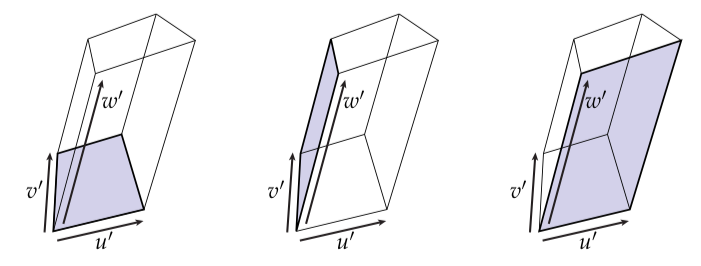\begin{aligned} \alpha \wedge \beta \wedge \gamma &= (u' \times v') \cdot w' \\ &= (v' \times w') \cdot u' \\ &= (w' \times u') \cdot v' \end{aligned}

$(\alpha \wedge \beta) \wedge \gamma = \alpha \wedge (\beta \wedge \gamma)$

• 反对称（Antisymmetry）： $\alpha \wedge \beta = (-1)^{kl}\beta \wedge \alpha$
• 结合律（Associativity）： $\alpha \wedge (\beta \wedge \gamma) = (\alpha \wedge \beta) \wedge \gamma$

• 分配律（Distributivity）： $\alpha \wedge (\beta + \gamma) = \alpha \wedge \beta + \alpha \wedge \gamma$

### 向量值形式

$\alpha \wedge \beta(u,v) \coloneqq \alpha(u)\beta(v) - \alpha(v)\beta(u)$

$\alpha \wedge \beta(u,v) = \alpha(u) \beta(v) - \alpha(v)\beta(u)$

## Hodge对偶性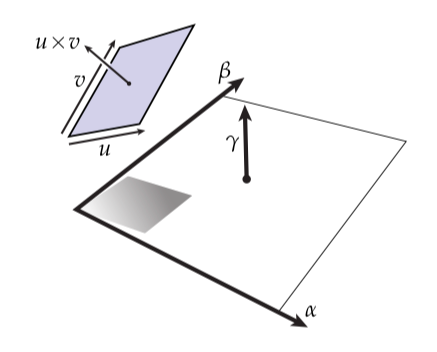$\gamma(u \times v) = \alpha \wedge \beta (u,v)$

### 微分形式和Hodge星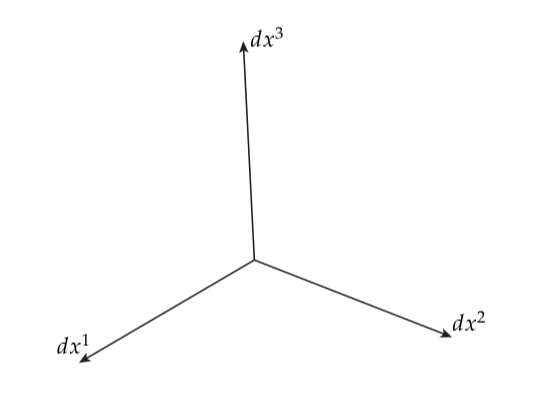$\alpha \wedge \beta = (\alpha_i dx^i) \wedge (\beta_j dx^j) = \alpha_i \beta_j dx^i \wedge dx^j$

$\begin{matrix} dx^1 \wedge dx^1 & dx^1 \wedge dx^2 & dx^1 \wedge dx^3 \\ dx^2 \wedge dx^1 & dx^2 \wedge dx^2 & dx^2 \wedge dx^3 \\ dx^3 \wedge dx^1 & dx^3 \wedge dx^2 & dx^3 \wedge dx^3 \end{matrix}$

$\begin{matrix} 0 & dx^1 \wedge dx^2 & -dx^3 \wedge dx^1 \\ - dx^1 \wedge dx^2 & 0 & dx^2 \wedge dx^3 \\ dx^3 \wedge dx^1 & - dx^2 \wedge dx^3 & 0 \end{matrix}$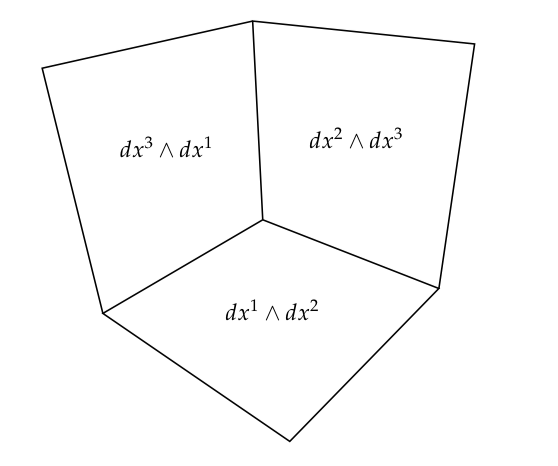$dx^1 \wedge dx^2 \wedge dx^3$

$dx^2 \wedge dx^3 \wedge dx^1 = - dx^2 \wedge dx^1 \wedge dx^3 = dx^1 \wedge dx^2 \wedge dx^3$

$dx^2 \wedge dx^1 \wedge dx^2 = -dx^2 \wedge dx^2 \wedge dx^1 = 0 \wedge dx^1 = 0$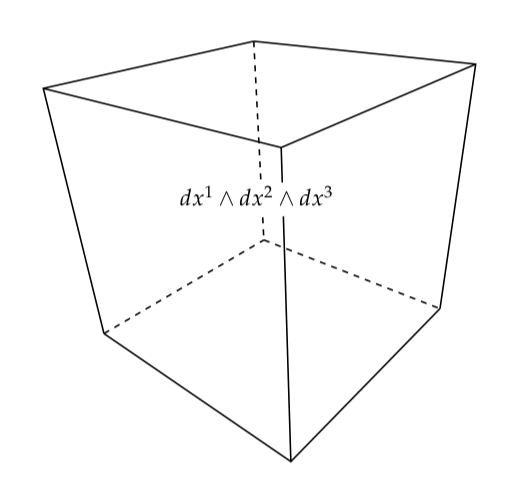• 0-型基： 1
• 1-型基： $dx^1, dx^2, dx^3$
• 2-型基： $dx^2 \wedge dx^3, dx^3 \wedge dx^1, dx^1 \wedge dx^2$
• 3-型基： $dx^1 \wedge dx^2 \wedge dx^3$

$\begin{pmatrix} n \\ k \end{pmatrix} = \frac{n!}{k!(n-k)!}$

（即“n中选k个”），由于我们想要获得k个不同的1-型基并且不关心顺序。这里有一个重要的恒等式就是

$\begin{pmatrix} n \\ k \end{pmatrix} = \begin{pmatrix} n \\ n-k \end{pmatrix}$

\begin{aligned} \star 1 &= dx^1 \wedge dx^2 \wedge dx^3 \\ \star dx^1 &= dx^2 \wedge dx^3 \\ \star dx^2 &= dx^3 \wedge dx^1 \\ \star dx^3 &= dx^1 \wedge dx^2 \\ \star(dx^1 \wedge dx^2) &= dx^3 \\ \star{dx^2 \wedge dx^3} &= dx^1 \\ \star(dx^3 \wedge dx^1) &= dx^2 \\ \star(dx^1 \wedge dx^2 \wedge dx^3) &= 1 \end{aligned}

$\star(dx^{i_1} \wedge dx^{i_2} \wedge \cdots \wedge dx^{i_k}) = dx^{i_{k+1}} \wedge dx^{i_{i+2}} \wedge \cdots \wedge dx^{i_n}$

### 体积形式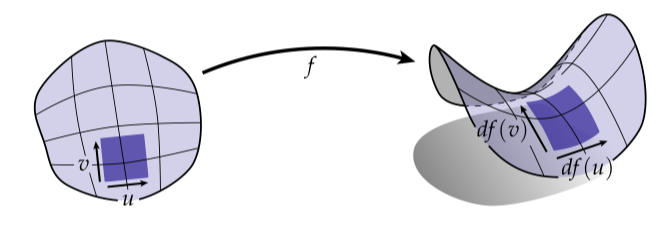$\omega \coloneqq \sqrt{\det(\mathsf{g})} dx^1 \wedge \cdots \wedge dx^n$

$\star 1 = \omega$

### 在k-型上的内积

$\alpha \wedge \star \beta = \langle \langle \alpha, \beta \rangle \rangle \omega$

$u \cdot v = \star (u^{\flat} \wedge \star v^{\flat})$

$u \times v = (\star(u^{\flat} \wedge v^{\flat}))^{\sharp}$

## 微分算子

$\nabla \coloneqq \left( \frac{\partial}{\partial x^1}, \cdots, \frac{\partial}{\partial x^n} \right)^T$

$\nabla \phi = \left( \frac{\partial f}{\partial x^1}, \cdots, \frac{\partial f}{\partial x^n} \right)^T$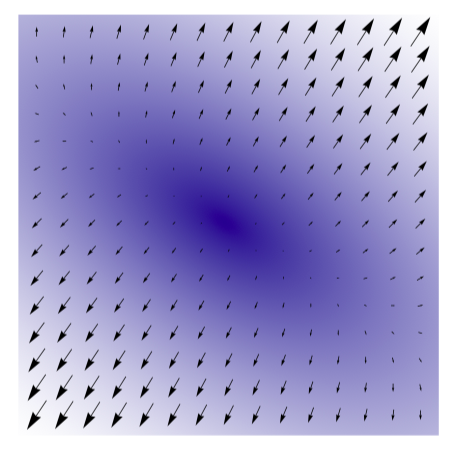$\nabla \cdot X = \frac{\partial X^1}{\partial x^1} + \cdots + \frac{\partial X^n}{\partial x^n}$

$\nabla \times X = \left( \frac{\partial X^3}{\partial x^2} - \frac{\partial X^2}{\partial x^3}, \frac{\partial X^1}{\partial x^3} - \frac{\partial X^3}{\partial x^1}, \frac{\partial X^2}{\partial x^1} - \frac{\partial X^1}{\partial x^2} \right)$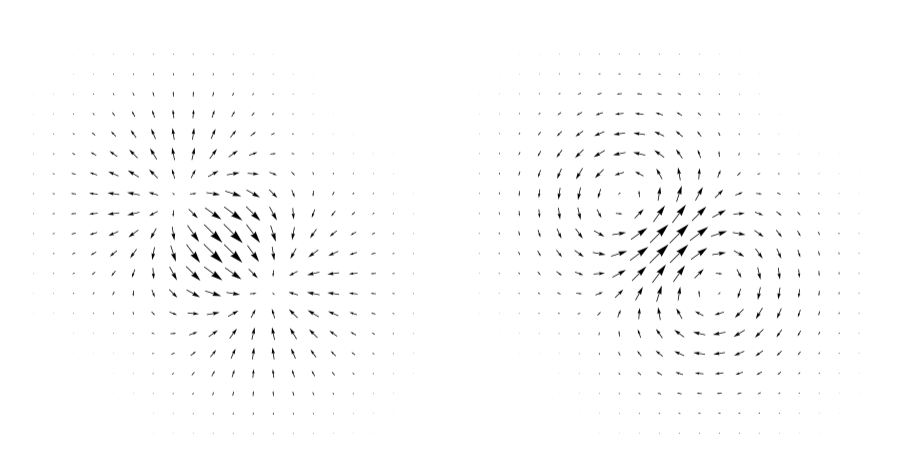（注意到在这个情况中一个场仅仅是另一个场90度的旋转！）使用这些类型的图像而不是使用上面那些丑陋的表达式对于理解散度、梯度和旋度是更加有用的。

### 考虑微分

$d \phi \coloneqq \frac{\partial \phi}{\partial x^1} dx^1 + \cdots + \frac{\partial \phi}{\partial x^n} dx^n$

$\nabla \phi = (d \phi)^{\sharp}$

### 方向导数

$df(u) = \frac{\partial f}{\partial x^1}u^1 + \cdots + \frac{\partial f}{\partial x^n}u^n$（将这个图和我们上面看到梯度的图比较一下。）一般的，$df(u)$表示$f$沿着方向$u$的方向导数（directional derivative）。换句话说，它告诉我们当我们在$u$方向上移动一小段距离时，$f$变化的有多快。再一次回到向量计算符号，我们有

$df(u) = u \cdot \nabla f$

### 外导数的性质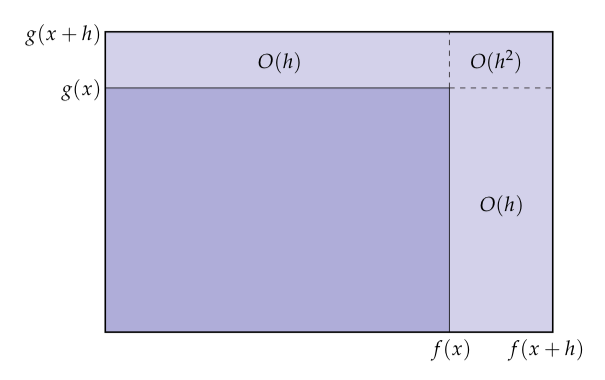$d(\alpha \wedge \beta) = d\alpha \wedge \beta + (-1)^k \alpha \wedge d(\beta)$

### 1-型的外导数

\begin{aligned} d \alpha &= d(\alpha_1 dx^1 + \alpha_2 dx^2 + \alpha_3 dx^3) \\ &= d(\alpha_1 dx^1) + d(\alpha_2 dx^2) + d(\alpha_3 dx^3) \end{aligned}

$d(\alpha_j \wedge dx^j) = (d \alpha_j) \wedge dx^j + \alpha_j \wedge \underbrace{(ddx^j)}_{=0} = \frac{\partial \alpha_j}{\partial x^i} dx^i \wedge dx^j$

\begin{aligned} dx &= \frac{\alpha_1}{\partial x^1} dx^1 \wedge dx^1 + \frac{\alpha_1}{\partial x^2} dx^2 \wedge dx^1 + \frac{\alpha_1}{\partial x^3} dx^3 \wedge dx^1 \\ &= \frac{\alpha_2}{\partial x^1} dx^1 \wedge dx^2 + \frac{\alpha_2}{\partial x^2} dx^2 \wedge dx^2 + \frac{\alpha_2}{\partial x^3} dx^3 \wedge dx^2 \\ &= \frac{\alpha_3}{\partial x^1} dx^1 \wedge dx^3 + \frac{\alpha_3}{\partial x^2} dx^2 \wedge dx^3 + \frac{\alpha_3}{\partial x^3} dx^3 \wedge dx^3 \end{aligned}

\begin{aligned} d \alpha &= \left( \frac{\partial \alpha_3}{\partial x^2} - \frac{\partial \alpha_2}{\partial x^3} \right) dx^2 \wedge dx^3 + \\ &= \left( \frac{\partial \alpha_1}{\partial x^3} - \frac{\partial \alpha_3}{\partial x^1} \right) dx^3 \wedge dx^1 + \\ &= \left( \frac{\partial \alpha_2}{\partial x^1} - \frac{\partial \alpha_1}{\partial x^2} \right) dx^1 \wedge dx^2 \end{aligned}

$\nabla \times X = \left( \star d X^{\flat} \right) ^{\sharp}$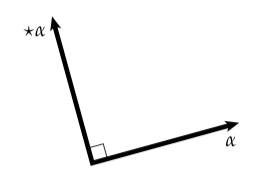$\nabla \cdot X = \star d \star X^{\flat}$

\begin{aligned} \star X^{\flat} &= \star (X_1 dx^1 + X_2 dx^2 + X_3 dx^3) \\ &= X_1 dx^2 \wedge dx^3 + X_2 dx^3 \wedge dx^1 + X_3 dx^1 \wedge dx^2 \end{aligned}

\begin{aligned} d \star X^{\flat} = &\frac{\partial X_1}{\partial x^1} dx^1 \wedge dx^2 \wedge dx^3 + \\ &\frac{\partial X_2}{\partial x^2}dx^2 \wedge dx^3 \wedge dx^1 + \\ &\frac{\partial X_3}{\partial x^3}dx^3 \wedge dx^1 \wedge dx^2 \end{aligned}

$d \star X^{\flat} = (\frac{\partial X_1}{\partial x^1} + \frac{\partial X_2}{\partial x^2} + \frac{\partial X_3}{\partial x^3})dx^1 \wedge dx^2 \wedge dx^3$

Hodge星最后的应用给我们带来了我们想要的散度

$\star d \star X^{\flat} = \frac{\partial X_1}{\partial x^1} + \frac{\partial X_2}{\partial x^2} + \frac{\partial X_3}{\partial x^3}$

\begin{aligned} \nabla \phi &= (d \phi)^{\sharp} \\ \nabla \times X &= \left( \star d X^{\flat} \right) ^{\sharp} \\ \nabla \cdot X &= \star d \star X^{\flat} \end{aligned}

### 拉普拉斯

$\Delta \coloneqq \nabla \cdot \nabla$

$\Delta \coloneqq \star d \star d + d \star d \star$

## 积分和Stokes‘定理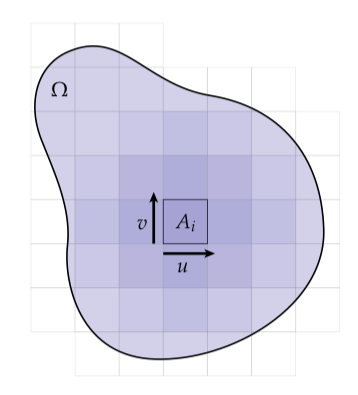$A_{\Omega} \approx \sum_i A_i$

$A_{\Omega} = \intop_{\Omega} dA$

$\intop _{\Omega} \phi dA = \intop _{\Omega} \phi dx^1 \wedge dx^2$

### 曲面上的积分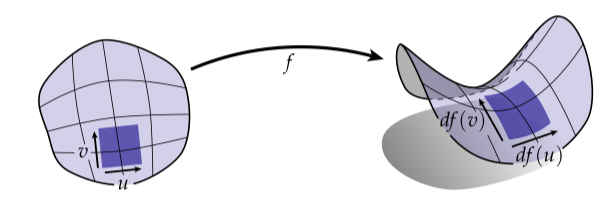$\omega = \sqrt{\det(\mathsf{g})} dx^1 \wedge dx^2$

$\intop_{\Omega} \phi \omega = \intop_{\Omega} \phi \sqrt{\det(\mathsf{g})} dx^1 \wedge dx^2$

$\intop_{\Omega} \phi a dx^1 \wedge dx^2$

### Stokes‘定理

$\intop_{\Omega} d\alpha = \intop_{\partial \Omega} \alpha$

### 散度定理

$\intop _{\Omega} \nabla \cdot X dA = \intop_{\partial \Omega} n \cdot X dl$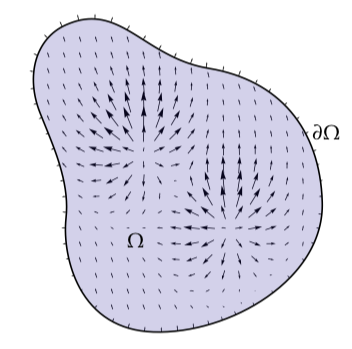$\intop_{\Omega} d \star X^{\flat} = \intop_{\partial \Omega} \star X^{\flat}$

### 微积分基本定理

$\intop ^b_a \frac{\partial \phi}{\partial x} dx = \phi(b) - \phi(a)$

$\intop_{[a,b]} d\phi = \intop_{\partial [a,b]}\phi$

## 离散外微积分### 离散微分形式

$\hat{\alpha}_e \coloneqq \intop_e \alpha$

$e$关联起来，其中（$\hat{\alpha}$）的意思是表示一个离散的量（不要和单位长度向量混淆）。

$\intop_e \alpha \approx |e| \left( \frac{1}{N} \sum^{N}_{i=1}\alpha_{p_i}(u) \right)$